Question

X-ray photons of wavelength 0.02480 nm are incident on a target and the Compton scattered photons are observed at 90 degrees.

(a) What is the wavelength of the scattered photon ?

(b) What is the momentum of the incident photon ?

(c) What is the kinetic energy of the scattered electron ?

(d) What is the momentum (magnitude and direction) of the scattered electron ?

1.Explanation:

(a) This problem can be solved by using the formula for Compton effect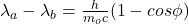where h is the planck constant, Φ is the angle of the scattered photon, m is the mass of the particle (in this case we take an electron) and c is the speed of ligth.

By taking the wavelength of the scattered photon and by replacing we have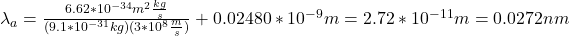(b)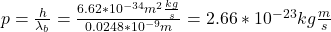(c)

The energy is conserved, hence we have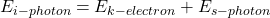that is, the sum of the kinetic energy of the scattered electron and the energy of the scattered photon is equal to the energy of the incident photon. By taking Ek we have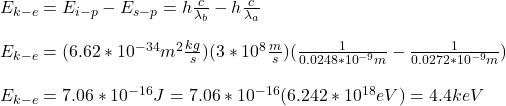(d)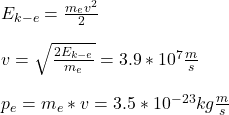I hope this is useful for you

regards